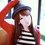# laws of motion ( to answer the problems )

## first law

vi = intial velocity vf = final velocity change of velocity = Vf - Vi acceleration = change of velocity / change of time acceleration = Vf-Vi ÷ t
at= vf - vi

## second law

displacement= average velocity X time average velocity = Vi + Vf ÷ 2 d = (Vi + Vf ÷ 2 ) X t WE SAY THAT 1st law
Vf= Vi + at WE WILL CANCEL vf and but the rule d = ( vi + vi + at ÷ 2) X t = 2vit + at with power 2 ÷2

## d = vit + half at with power two

3rd law ---------- NOTE : ^ this mean with power too ok vf = vi+at by squaring vf^ = ( vi + at )^ vf ^ = vi^ + 2viat + a^t^ vf^-vi^=2viat+a^t^ = 2a(vit + half at^ )

THEN THE RULE IS note :^ this mean with power two 1) Vi + at = Vf first law 2) d = vit + half at ^ second law 3) Vf^- Vi^= 2ad third lawNote by Nawara Elhussein
5 years, 8 months ago

This discussion board is a place to discuss our Daily Challenges and the math and science related to those challenges. Explanations are more than just a solution — they should explain the steps and thinking strategies that you used to obtain the solution. Comments should further the discussion of math and science.

When posting on Brilliant:

• Use the emojis to react to an explanation, whether you're congratulating a job well done , or just really confused .
• Ask specific questions about the challenge or the steps in somebody's explanation. Well-posed questions can add a lot to the discussion, but posting "I don't understand!" doesn't help anyone.
• Try to contribute something new to the discussion, whether it is an extension, generalization or other idea related to the challenge.

MarkdownAppears as
*italics* or _italics_ italics
**bold** or __bold__ bold
- bulleted- list
• bulleted
• list
1. numbered2. list
1. numbered
2. list
Note: you must add a full line of space before and after lists for them to show up correctly
paragraph 1paragraph 2

paragraph 1

paragraph 2

[example link](https://brilliant.org)example link
> This is a quote
This is a quote
    # I indented these lines
# 4 spaces, and now they show
# up as a code block.

print "hello world"
# I indented these lines
# 4 spaces, and now they show
# up as a code block.

print "hello world"
MathAppears as
Remember to wrap math in $$ ... $$ or $ ... $ to ensure proper formatting.
2 \times 3 $2 \times 3$
2^{34} $2^{34}$
a_{i-1} $a_{i-1}$
\frac{2}{3} $\frac{2}{3}$
\sqrt{2} $\sqrt{2}$
\sum_{i=1}^3 $\sum_{i=1}^3$
\sin \theta $\sin \theta$
\boxed{123} $\boxed{123}$

Sort by:

Try to code in Latex.

- 5 years, 8 months ago

Thanks!

- 5 years, 8 months ago